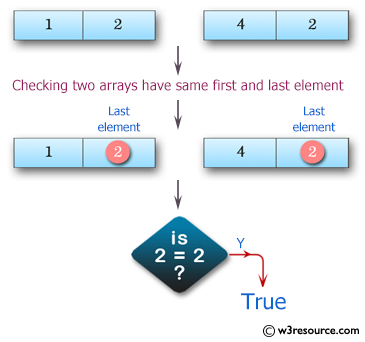﻿ Swift Array Programming Exercise: Test if two given arrays of integers have the same first or last element - w3resource# Swift Array Programming Exercises: Test if two given arrays of integers have the same first or last element

## Swift Array Programming: Exercise-3 with Solution

Write a Swift program to test if two given arrays of integers have the same first or last element. Both arrays length must be 1 or more.

Pictorial Presentation:Sample Solution:

Swift Code:

``````func first_last(_ a: [Int], _ b: [Int]) -> Bool {
guard a.count > 0, b.count > 0 else
{
return false
}

if a.first == b.first || a.last == b.last
{
return true
}
else
{
return false
}
}
print(first_last([1, 2, 3], [5, 1]))
print(first_last([1, 2], [4, 2]))
print(first_last([1, 2, 3, 1], [-1, 3, 5]))
```
```

Sample Output:

```false
true
false
```

Swift Programming Code Editor:

Improve this sample solution and post your code through Disqus

What is the difficulty level of this exercise?

﻿